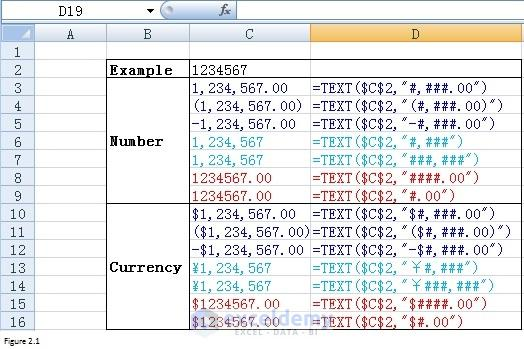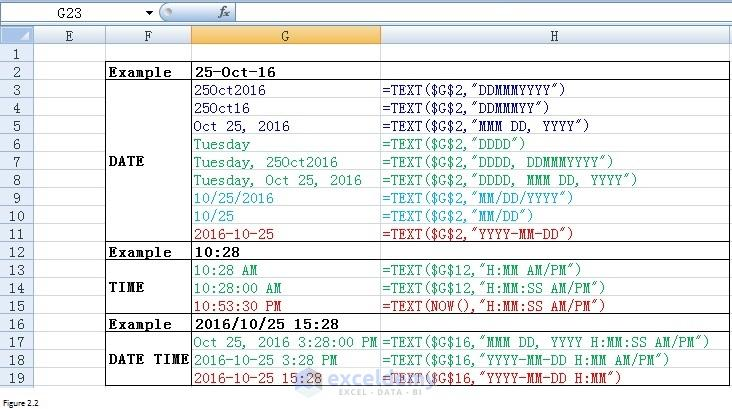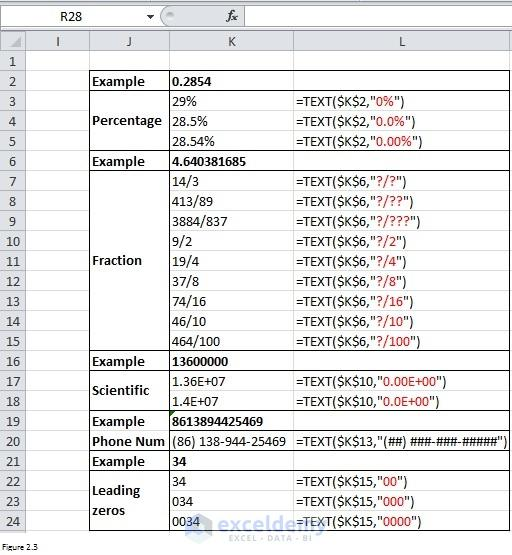Using FORMAT & TEXT Functions in Excel (68 Examples)

In this article, you will learn how to use Excel format function and text function with 68 examples. These examples will clear your idea about these two functions.

Sometimes, we need to format or manipulate data the way what we want. Here is what we generally do. If you right-click on the worksheet, the context menus will be prompted. By clicking on Format Cells in context menus, we can open Format Cells dialog box. From Format Cells dialog box, we can see that the way number, currency, date, time or percentage will be displayed can be changed by making an appropriate selection.Figure 1

However, there are other methods that are available. Today I will show you how to format convert number, date, time, currency and so on using two functions: TEXT function and Format function. TEXT function is a worksheet function which you can use in worksheet while Format function can only be used in VBA code.

TEXT Function

Syntax of TEXT function

Text function can convert a value to text with a specific format. Here is the syntax for TEXT function:

 TEXT(value, format) Value The value to convert to text Format The format used to display the result

Format Number or Currency using TEXT function

Figure 2.1 shows how to use TEXT function to format number or currency. Values in range C3:C16 are the results returned from formula listed in range D3:D16. From cells C3 through D5, we can not only add a separator or minus sign into number but also put number into parentheses. Plus, we can also determine decimal places. Range C6:D7 tells that format “#,###” is enough to enable us to add a thousand separator. It is not necessary to use a format such as “###,###”. Format “#” can be used when you don’t want to display thousand separators per range C8:D9. Range C10:d16 shows how to display currency. “\$” is for dollar while “￥” is for Yuan which is the currency for China. You can use other currency symbols too as long as you put them at the beginning of the format.Figure 2.1

Format Date or Time using TEXT function

Range G3:H11 in Figure 2.2 shows how to format date. And you can see that there are a lot of ways to display the date as you wish. Range G13:H15 shows how to format time. Sometimes, we may need to display date and time together. Range G17:H19 presents three ways to format DateTime value. In fact, all combinations of date format (in G3:H11) and time format (in G13:H15) can be used to format DateTime value. You can try by yourself.Figure 2.2

Format Percentage, Fraction, Scientific number or telephone number using TEXT function

From Figure 1, we can see that TEXT function can also be used to format fraction, percentage, scientific number, etc. For example, range K2:L5 shows that 0.2854 can be displayed with a percent symbol. And you can even determine the number of decimal places by applying TEXT function. As for fraction, range K7:L9 shows that you can determine the number of digits in the numerator. You can even determine what the numerator is per cells from K10 through L15. Range K16:L20 presents you how to format scientific number or telephone number. The last point that I want to show you is how to add leading zeros. If our test number in cell K21 is 340 instead of 34, the number in cell K24 will be “0340”.Figure 2.3

Using Excel FORMAT Function

FORMAT function is a VBA function which you can use in macro code. I have to remind you that FORMAT function cannot be used in the worksheet and you should always enter it into Visual Basic Editor when using it.

Syntax of FORMAT function

The syntax for FORMAT function is similar to that for TEXT function. But the format here is fixed. It is not as flexible as that for TEXT function. Below table shows you the format and the corresponding explanation.

 Format Explanation General Number Displays a number without thousand separators. Currency Displays thousand separators as well as two decimal places. Fixed Displays at least one digit to the left of the decimal place and two digits to the right of the decimal place. Standard Displays the thousand separators, at least one digit to the left of the decimal place, and two digits to the right of the decimal place. Percent Displays a percent value – that is, a number multiplied by 100 with a percent sign. Displays two digits to the right of the decimal place. Scientific Scientific notation. Yes/No Displays No if the number is 0. Displays Yes if the number is not 0. True/False Displays False if the number is 0. Displays True if the number is not 0. On/Off Displays Off if the number is 0. Displays On is the number is not 0. General Date Displays date based on your system settings Long Date Displays date based on your system’s long date setting Medium Date Displays date based on your system’s medium date setting Short Date Displays date based on your system’s short date setting Long Time Displays time based on your system’s long time setting Medium Time Displays time based on your system’s medium time setting Short Time Displays time based on your system’s short time setting

Code to setup example

Here shows you how to use FORMAT function in VBA.

 Source code```Sub Format_func()

Worksheets(2).Activate

'Number

Cells(2, 3) = 123456

Cells(3, 3) = Format(Cells(2, 3), "General Number")

Cells(4, 3) = Format(Cells(2, 3), "Currency")

Cells(5, 3) = Format(Cells(2, 3), "Fixed")

Cells(6, 3) = Format(Cells(2, 3), "Standard")

Cells(7, 3) = Format(Cells(2, 3), "Scientific")

'Percentage

Cells(8, 3) = 0.88

Cells(9, 3) = Format(Cells(8, 3), "Percent")

'Logic

Cells(11, 3) = 2

Cells(12, 3) = Format(Cells(11, 3), "Yes/No")

Cells(13, 3) = Format(Cells(11, 3), "True/False")

Cells(14, 3) = Format(Cells(11, 3), "On/Off")

Cells(15, 3) = 0

Cells(16, 3) = Format(Cells(15, 3), "Yes/No")

Cells(17, 3) = Format(Cells(15, 3), "True/False")

Cells(18, 3) = Format(Cells(15, 3), "On/Off")

'Date

Cells(2, 7) = "Sep 3, 2003"

Cells(3, 7) = Format(Cells(2, 7), "General Date")

Cells(4, 7) = Format(Cells(2, 7), "Long Date")

Cells(5, 7) = Format(Cells(2, 7), "Medium Date")

Cells(6, 7) = Format(Cells(2, 7), "Short Date")

'Time

Cells(8, 7) = "15:25"

Cells(9, 7) = Format(Cells(8, 7), "Long Time")

Cells(10, 7) = Format(Cells(8, 7), "Medium Time")

Cells(11, 7) = Format(Cells(8, 7), "Short Time")

End Sub```

Results returned by FORMAT function

Column C shows results returned by FORMAT function in column D. Similarly, column G presents what returned by FORMAT function in column H. Values in cells C12 through C14 will be the same as that in Figure 3 as long as the value in cell C11 does not equal to 0. This is inconsistent with the explanations in above table. You can have a try on your own.

Reminder

FORMAT function cannot be applied in worksheet and TEXT function cannot be used in VBA. Figure 4 illustrates that something like “#NAME?” will be returned if you apply FORMAT function in a worksheet. And Excel will give you a prompted message like “Sub or function not defined” if you use TEXT function in VBA.

Text-and-Format-Excel-functions.xlsm

Zhiping Yan

I am from China and this photo was taken in a classical garden. There are many similar gardens in China, attracting a lot of visitors every year, especially in spring and summer. I was major in Biotechnology. But I took a job as an SAS programmer because I prefer programming. Besides SAS, I also learned Excel VBA in my spare time. It is fantastic to be able to manipulate data, files and even to interact with the internet via programming. This will save me a lot of time. I am keen to learn new things.

Roger Gruenenfelder December 14, 2016 at 7:31 AM

Good day. Is it right that once I put a number or date in a Text format, I am unable to calculate with it ?

Kawser December 14, 2016 at 9:37 AM

Yes, when a number or date is placed in a cell in Text format, you cannot calculate with them.

Roger Gruenenfelder December 15, 2016 at 11:29 PM

Thanks. I have another question.
Let’s say I have a problem within an existing sheet or workbook and try to ask you for a solution by texting you (f.e. in this comment space). This might be unconvincing for you, because, either I don’t know how to formulate my problem or you can’t see the building of my sheet/formulas etc.
Is there a way to send you example files, where you can go through and see where I made a ridiculous mistake and you have the power to help me with your immense knowledge ?